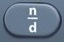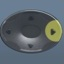# Equations

This = That

Basics
An algebraic equation is a particular type of algebraic expression using the = sign.

An equation means  This = That

Whatever is on the left side of an equation = Whatever is on the right side of an equation

Whatever is done to the left side of an equation (add, subtract, multiply, divide) =
Whatever is done to the right side of an equation (add, subtract, multiply, divide)

A question involving an equation often means solving for the numerical value of a variable.

When solving:
• It is helpful to isolate the variable in question on the left side of the equation.
• It is helpful to perform a mathematical operation that is the opposite of the equation’s original mathematical operation.
• It is helpful to know your multiplication and division tables.  However, the TI-30XS MultiView Scientific Calculator can be used as needed.
• For the sake of order of operations, it is better to set up division language in$\bf\displaystyle\frac{x}{y}$ form rather than x÷y form.

Question
Given x + 3 = 8, solve for x.

x = 5

x + 3 = 8

x + 3 – 3 = 8 – 3
• 3 is subtracted from each side of = sign
• subtraction is the opposite of addition

x = 5
•  3 – 3 is the same as 0, so it disappears
•  8 – 3 is the same as 5
• x has been isolated on the left side of = sign

Question
Given x – 3 = 8, solve for x.

x = 11

x – 3 = 8

x – 3 + 3 = 8 + 3
• 3 is added to each side of = sign
• addition is the opposite of subtraction

x = 11
•  -3 + 3 is the same as 0, so it disappears
•  8 + 3 is the same as 11
• x has been isolated on the left side of = sign

Question
Given 3y =27, solve for y.

y = 9

3y = 27$\bf\displaystyle\frac{3y}{3}$ =$\bf\displaystyle\frac{27}{3}$
• each side of = sign is divided by 3
• division is the opposite of multiplication

y = 9
•$\bf\displaystyle\frac{3y}{3}$ is the same as 1y which is the same as y$\bf\displaystyle\frac{27}{3}$ is the same as 9
• y has been isolated on the left side of = sign

Question
Given$\bf\displaystyle\frac{y}{5}$ = 4, solve for y.

y = 20$\bf\displaystyle\frac{y}{5}$ = 4$\bf\displaystyle\frac{5y}{5}$ = 5·4
• each side of = sign is multiplied by 5
• multiplication is the opposite of division

y = 20
•$\bf\displaystyle\frac{5y}{5}$ is the same as 1y which is the same as y
• 5·4 is the same as 20
• y has been isolated on the left side of = sign

Question
Given 5y + 6 = 26, solve for y.

y = 4

5y + 6 = 26

5y + 6 – 6 = 26 – 6
• 6 is subtracted from each side of = sign
• subtraction is the opposite of addition

5y = 20
• 6 – 6 is the same as 0, so it disappears
• 26 – 6 is the same as 20$\bf\displaystyle\frac{5y}{5}$ =$\bf\displaystyle\frac{20}{5}$
• each side of = sign is divided by 5

• division is the opposite of multiplication

y = 4
•$\bf\displaystyle\frac{5y}{5}$ is the same as 1y which is the same as y$\bf\displaystyle\frac{20}{5}$ is the same as 4
• y has been isolated on the left side of = sign

Question
Given$\bf\displaystyle\frac{y}{7}$ – 3 = 5, solve for y.

y = 56$\bf\displaystyle\frac{y}{7}$ – 3 = 5$\bf\displaystyle\frac{y}{7}$ – 3 + 3 = 5 + 3
• 3 is added to each side of = sign
• addition is the opposite of subtraction$\bf\displaystyle\frac{y}{7}$ = 8
• -3 + 3 is the same as 0, so it disappears
• 5 + 3 is the same as 8$\bf\displaystyle\frac{7y}{7}$ = 7·8
• each side of = sign is multiplied by 7
• multiplication is the opposite of division

y = 56
•$\bf\displaystyle\frac{7y}{7}$ is the same as 1y which is the same as y
• 7·8 is the same as 56
• y has been isolated on the left side of = sign

Input Display Commentblinker clears screen
5 + 3 5+3 addition on right side of =8
7 × 8 7*8 multiplication on right side of =56 Answer
###### Equation

Question
Given 16y + 6 = 126, solve for y in decimal form.

y = 7.5

16y + 6 = 126

16y + 6 – 6 = 126 – 6
• 6 is subtracted from each side of = sign
• subtraction is the opposite of addition

16y = 120
• 6 – 6 is the same as 0, so it disappears
• 126 – 6 is the same as 120$\bf\displaystyle\frac{16y}{16}$ =$\bf\displaystyle\frac{120}{16}$
• each side of = sign is divided by 16
• division is the opposite of multiplication

y = 7.5
•$\bf\displaystyle\frac{16y}{16}$ is the same as 1y which is the same as y$\bf\displaystyle\frac{120}{16}$ is the same as$\bf\displaystyle\frac{15}{2}$ which is the same as 7.5 (after toggle)
• y has been isolated on the left side of = sign

Input Display Commentblinker clears screen
126 – 6 126-6 subtraction on right side of =12012016$\bf\displaystyle\frac{120}{16}$ division on right side of =$\bf\displaystyle\frac{15}{2}$7.5 Answer
(Toggle)
###### Equation

Question
Five collectors threw away 4 worthless stamps at a trade show.  Each collector ended up with 26 stamps.

Given the equation$\bf\displaystyle\frac{s}{5}$ – 4 = 26, how many total stamps s were originally distributed among the 5 collectors?

s = 150$\bf\displaystyle\frac{s}{5}$ – 4 = 26$\bf\displaystyle\frac{s}{5}$ – 4 + 4 = 26 + 4
• 4 is added to each side of = sign
• addition is the opposite of subtraction$\bf\displaystyle\frac{s}{5}$ = 30
• -4 + 4 is the same as 0, so it disappears
• 26 + 4 is the same as 30$\bf\displaystyle\frac{5s}{5}$ = 5·30
• each side of = sign is multiplied by 5
• multiplication is the opposite of division

s = 150
•$\bf\displaystyle\frac{5s}{5}$ is the same as 1s which is the same as s
• 5·30 is the same as 150
• s has been isolated on the left side of = sign

Input Display Commentblinker clears screen
26 + 4 26+4 addition on right side of =30
5 × 30 5*30 multiplication on right side of =130 Answer
###### Equation

Question
A landscaping crew planted 12 trees an hour plus an additional 7 trees for a total of 223 trees.

Given the equation 12h + 7 = 223, how many hours h did the crew spend planting?

h = 18

12h + 7 = 223

12h + 7 – 7  =  223 – 7
• 7 is subtracted from each side of = sign
• subtraction is the opposite of addition

12h = 216
• 7 – 7 is the same as 0, so it disappears
• 223 – 7 is the same as 216$\bf\displaystyle\frac{12h}{12}$ =$\bf\displaystyle\frac{216}{12}$
• each side of = sign is divided by 12
• division is the opposite of multiplication

h = 18
•$\bf\displaystyle\frac{12h}{12}$ is the same as 1h which is the same as h$\bf\displaystyle\frac{216}{12}$ is the same as 18
• h has been isolated on the left side of = sign

Input Display Commentblinker clears screen
223 – 7 223-7 subtraction on right side of =21621612$\bf\displaystyle\frac{216}{12}$ division on right side of =18 Answer
###### Equation

Practice – Questions
1.  Given x + 4 = 18, solve for x.

2.  Given x – 4 = 12, solve for x.

3.  Given$\bf\displaystyle\frac{y}{5}$ – 4 = 3, solve for y.

4.  Given 16y + 8 = 146, solve for y in decimal form.

5.  Five hobbyists threw away 3 worthless baseball cards at a trade show. Each hobbyist ended up with 22 baseball cards.  Given the equation$\bf\displaystyle\frac{c}{5}$ – 3 = 22, how many total cards c were originally distributed among the 5 hobbyists?

6.  Four regular umpires umpired 39 games apiece during the baseball season.  Two extra games were umpired by substitute umpires.  Given the equation$\bf\displaystyle\frac{g}{4}$ + 2 = 39, how many total games g were umpired?

7.  A dog collected 15 good bones a day plus 8 bones unworthy of keeping for a total of 158 bones.  Given the equation 15d + 8 = 158, how many days d did the dog spend collecting bones?

8.  A teacher concocted 10 original test questions an hour minus 5 duplicate questions for a total of 125 test questions.  Given the equation 10h – 5 = 125, how many hours h did the teacher spend concocting test questions?

9.  A bird intended to fly 979 miles.  The bird covered 14 miles an hour before stopping 43 miles short to swim in a pond.  Given the equation 14h – 43 = 979, how many hours h did it take the bird to reach the pond?

10.  A shopper spent $96.00 at the mall, including$5.00 for parking.  The shopper spent \$7.00 apiece on accessories.  Given the equation 7a + 5 = 96, how many accessories a did the shopper buy?

1.  x = 14

2.  x = 16

3.  y = 35

4.  y = 8.625

5.  c = 125

6.  g = 148

7.  d = 10

8.  h = 13

9.  h = 73

10.  a = 13

## One thought on “Equations”

1.consentido83 says:

very good I LIKED!!